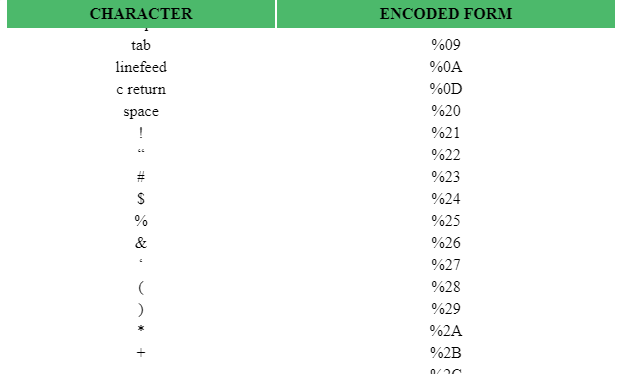# How to create sticky table headers in Chrome ?

The headers of a table remain fixed at the top of the screen while you scroll down through the table are called the sticky table headers. They are used to help the viewers to know the purpose of data in the columns when the original headers are lost from the view. It eliminates the inconvenience of scrolling up and down the table to get the reference of the data being read. Here, we will be seeing how to create sticky table headers in a table.

Approach: The approach is to set the position property as sticky on the table headers so that they remain fixed at the top while scrolling down the table.

Example:

 ` ` `<``html``> ` ` `  `<``head``> ` `    ``<``title``> ` `        ``How to create sticky table  ` `        ``headers In Chrome? ` `    `` ` `     `  `    ``<``style``> ` `        ``th { ` `            ``padding: 5px; ` `            ``background-color: #4cb96b; ` `            ``position: -webkit-sticky; ` `            ``position: sticky; ` `            ``top: 0; ` `        ``} ` `    `` ` ` ` ` `  `<``body``> ` `    ``<``p``> ` `        ``Below is a table which has  ` `        ``sticky table headers ` `    `` ` ` `  `    ``<``table` `width``=``"100%"``> ` `        ``<``thead``> ` `            ``<``th``>CHARACTER ` `            ``<``th``>ENCODED FORM ` `        `` ` `        ``<``tbody` `style``=``"text-align: center;"``> ` `            ``<``tr``> ` `                ``<``td``>backspace ` `                ``<``td``>%08 ` `            `` ` `            ``<``tr``> ` `                ``<``td``>tab ` `                ``<``td``>%09 ` `            `` ` `            ``<``tr``> ` `                ``<``td``>linefeed ` `                ``<``td``>%0A ` `            `` ` `            ``<``tr``> ` `                ``<``td``>c return ` `                ``<``td``>%0D ` `            `` ` `            ``<``tr``> ` `                ``<``td``>space ` `                ``<``td``>%20 ` `            `` ` `            ``<``tr``> ` `                ``<``td``>! ` `                ``<``td``>%21 ` `            `` ` `            ``<``tr``> ` `                ``<``td``>“ ` `                ``<``td``>%22 ` `            `` ` `            ``<``tr``> ` `                ``<``td``># ` `                ``<``td``>%23 ` `            `` ` `            ``<``tr``> ` `                ``<``td``>\$ ` `                ``<``td``>%24 ` `            `` ` `            ``<``tr``> ` `                ``<``td``>% ` `                ``<``td``>%25 ` `            `` ` `            ``<``tr``> ` `                ``<``td``>& ` `                ``<``td``>%26 ` `            `` ` `            ``<``tr``> ` `                ``<``td``>‘ ` `                ``<``td``>%27 ` `            `` ` `            ``<``tr``> ` `                ``<``td``>( ` `                ``<``td``>%28 ` `            `` ` `            ``<``tr``> ` `                ``<``td``>) ` `                ``<``td``>%29 ` `            `` ` `            ``<``tr``> ` `                ``<``td``>* ` `                ``<``td``>%2A ` `            `` ` `            ``<``tr``> ` `                ``<``td``>+ ` `                ``<``td``>%2B ` `            `` ` `            ``<``tr``> ` `                ``<``td``>, ` `                ``<``td``>%2C ` `            `` ` `            ``<``tr``> ` `                ``<``td``>– ` `                ``<``td``>%2D ` `            `` ` `            ``<``tr``> ` `                ``<``td``>. ` `                ``<``td``>%2E ` `            `` ` `            ``<``tr``> ` `                ``<``td``>/ ` `                ``<``td``>%2F ` `            `` ` `            ``<``tr``> ` `                ``<``td``>0 ` `                ``<``td``>%30 ` `            `` ` `            ``<``tr``> ` `                ``<``td``>1 ` `                ``<``td``>%31 ` `            `` ` `            ``<``tr``> ` `                ``<``td``>2 ` `                ``<``td``>%32 ` `            `` ` `            ``<``tr``> ` `                ``<``td``>3 ` `                ``<``td``>%33 ` `            `` ` `            ``<``tr``> ` `                ``<``td``>4 ` `                ``<``td``>%34 ` `            `` ` `            ``<``tr``> ` `                ``<``td``>5 ` `                ``<``td``>%35 ` `            `` ` `            ``<``tr``> ` `                ``<``td``>6 ` `                ``<``td``>%36 ` `            `` ` `            ``<``tr``> ` `                ``<``td``>7 ` `                ``<``td``>%37 ` `            `` ` `            ``<``tr``> ` `                ``<``td``>8 ` `                ``<``td``>%38 ` `            `` ` `            ``<``tr``> ` `                ``<``td``>9 ` `                ``<``td``>%39 ` `            `` ` `        `` ` `    `` ` ` ` ` `  ` `

Output:

``````

Explanation:
position: sticky attribute is used to make the header fixed relative to the table body. Remember sticky is not applicable on <thead> or <tr> tags but can be used with <th>. So we style the position of <th> as sticky and set it a dark background color otherwise the transparency would have made the other data of the table visible behind the headers while scrolling down. The top attribute is set as 0 to make the header fixed at the top.My Personal Notes arrow_drop_upCheck out this Author's contributed articles.

If you like GeeksforGeeks and would like to contribute, you can also write an article using contribute.geeksforgeeks.org or mail your article to contribute@geeksforgeeks.org. See your article appearing on the GeeksforGeeks main page and help other Geeks.

Please Improve this article if you find anything incorrect by clicking on the "Improve Article" button below.